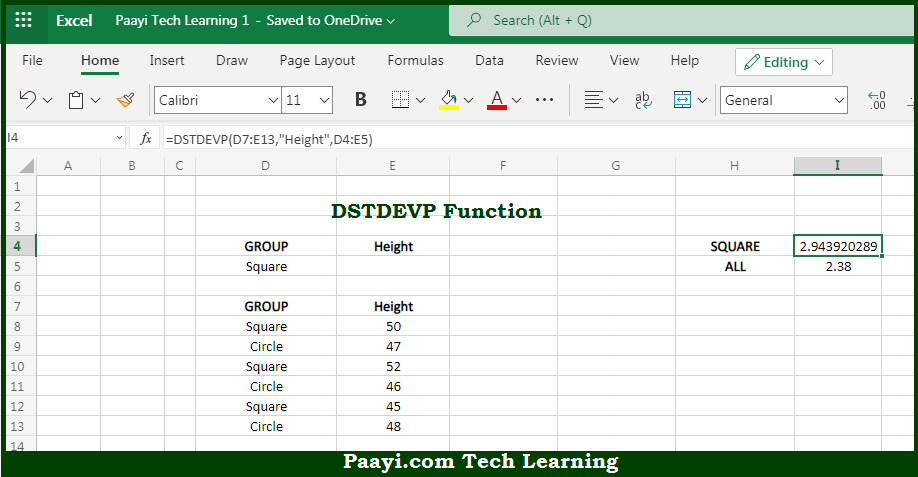# Learn How to Use Microsoft Excel DSTDEVP Function

Written by | 0 Comments | 642 Views

In this article, you will learn how to use the Microsoft Excel DSTDEVP function and its prime function in Microsoft Excel. You will also get to know the Microsoft Excel DSTDEVP function return value and syntax with the help of some examples.

Microsoft Excel DSTDEVP Function

The main purpose of the Microsoft Excel DSTDEVP function is to get the standard deviation of population from the matching records in the database. That implies, with the help of the DSTDEVP function you can able to return the standard deviation of an entire population extracted from records that match the given criteria. You can use the DSTDEVP function in case data If data represents the entire population. So, with the help of the DSTDEVP function, you can able to get the standard deviation of the entire population from the matching records in the database.

Return Value of DSTDEVP Function

The return value will be the calculated standard deviation.

Syntax of DSTDEVP Function

=DSTDEVP(database, field, criteria)

Where the arguments:

• database: This is the database range including headers.
• field: This is a field name or index to count optional.
• criteria: This is the criteria range including headers.

How to Use Microsoft Excel DSTDEVP Function?So we know that Microsoft Excel DSTDEVP function you can able to get the standard deviation of population from the matching records in the database. That implies, with the help of the DSTDEVP function you can able to return the standard deviation of an entire population extracted from records that match the given criteria. You can use the DSTDEVP function in case data If data represents the entire population. So, with the help of the DSTDEVP function, you can able to get the standard deviation of the entire population from the matching records in the database.